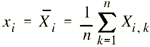Evaluating uncertainty components: Type A

A Type A evaluation of standard uncertainty may be based on any valid statistical method for treating data. Examples are calculating the standard deviation of the mean of a series of independent observations; using the method of least squares to fit a curve to data in order to estimate the parameters of the curve and their standard deviations; and carrying out an analysis of variance (ANOVA) in order to identify and quantify random effects in certain kinds of measurements.

Mean and standard deviation

As an example of a Type A evaluation, consider an input quantity Xi whose value is estimated from n independent observations Xi ,k of Xi obtained under the same conditions of measurement. In this case the input estimate xi is usually the sample mean(4)

and the standard uncertainty u(xi) to be associated with xi is the estimated standard deviation of the mean(5)

Continue
to Evaluating uncertainty components: Type B